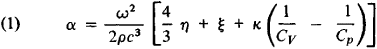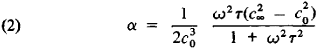# Absorption of Sound

The following article is from The Great Soviet Encyclopedia (1979). It might be outdated or ideologically biased.

## Absorption of Sound

the conversion of the energy of a sound wave into other forms of energy, particularly heat. Sound absorption is characterized by the amplitude absorption coefficient a, a quantity defined as the reciprocal of the distance at which the sound wave amplitude is reduced to 1/e = 1/2.718 of its initial value. The coefficient α is expressed in cm-1—that is, in nepers per cm—or in decibels (dB) per m (1 dB/m = 1.15 × 10-3 cm-1). The absorption of sound is also characterized by the loss factor ∊ = αλ/π, where λ is the wavelength of the sound, and the quality factor Q = 1/∊. The quantity αλ is known as the logarithmic damping decrement.

When sound propagates in a medium possessing viscosity and thermal conductivity,where ρ is the density of the medium, c is the speed of sound in the medium, ω is the angular frequency of the sound wave, η and ξ are, respectively, the coefficients of shear and bulk viscosity, κ is the coefficient of thermal conductivity, and Cp and Cv are the specific heats of the medium at constant pressure and at constant volume, respectively. If none of the coefficients η, ξ, or κ are frequency-dependent, a situation that is often the case in practice, then α ~ ω2. If the equilibrium state of the medium is disturbed during the passage of the sound, the absorption is substantially higher than that given by equation (1). Such absorption is termed relaxation sound absorption and is described by the formulawhere τ is the relaxation time and c0 and c are, respectively, the speed of sound when ωτ ≪ 1 and when ωτ > 1. The absorption in this case is accompanied by dispersion of the sound. The quantity α/f2, where f = ω/2π, is for a given substance the characteristic that determines the absorption of sound. It is generally smaller in liquids than in gases, and for longitudinal waves it is smaller in solids than in liquids.

Sound absorption in gases is a function of the gas pressure, and rarefaction of the gas is equivalent to an increase in frequency. The contributions of thermal conductivity and shear viscosity in gases to absorption are of the same order of magnitude. In liquids the absorption of sound is determined primarily by viscosity, and the contribution of thermal conductivity is negligibly small.

Bulk viscosity and relaxation processes play an important role in sound absorption in the majority of liquids. The relaxation frequency in liquids—that is, the quantity ωr = 1/τ—is generally very high, and the relaxation region lies in the high ultrasonic and hypersonic frequency ranges. The absorption coefficient is usually strongly dependent on temperature and on the presence of impurities.

Sound absorption in solids is chiefly a function of internal friction and the thermal conductivity of the medium, but at high frequencies and low temperatures it is dependent on various interaction processes between the sound and internal excitations in the solid—such as phonons, electrons, and spin waves. The magnitude of the absorption in a solid depends on several factors: the crystalline state of the substance (in single crystals absorption is usually less than in polycrystals); the presence of defects, impurities, and dislocations; and the previous treatment the material has undergone. In metals that have been given a preliminary heat treatment and have undergone such processes as forging and rolling, the absorption is often dependent on the amplitude of the sound. In many solids, when the frequency is not very high, α ∼ ω; as a result, the value of the quality factor is independent of the frequency and can be used to characterize the material’s losses. The lowest absorption at room temperatures has been observed in certain dielectrics, such as topaz, beryl, and yttrium-iron garnet, where α ∼ 15 dB/cm at f = 9 gigahertz. The absorption is always greater in metals and semiconductors than in dielectrics because of the extra absorption associated with the interaction between the sound and the conduction electrons. In semiconductors this interaction can under certain conditions result in “negative absorption,” that is, in amplification of the sound. As the temperature rises, the absorption of sound generally increases.

The presence of inhomogeneities in a medium causes the absorption to increase. Since various porous and fibrous substances exhibit high absorption, they can be used for damping and soundproofing.

### REFERENCES

Bergmann, L. Ul’trazvuk i ego primenenie v nauke i tekhnike, 2nd ed. Moscow, 1957. (Translated from German.)
Mikhailov, I. G., V. A. Solov’ev, and Iu. P. Syrnikov. Osnovy molekuliarnoi akustiki. Moscow, 1964.
Fizicheskaia akustika, vol. 2, part A, vol. 3, part B. Moscow, 1968-69. Vol. 7. Moscow, 1974. Edited by W. Mason. (Translated from English.)
Truell, R., C. Elbaum, and B. Chick. Ul’trazvukovye metody v fizike tverdogo tela. Moscow, 1972. (Translated from English.)

A. L. POLIAKOVA

References in periodicals archive ?
Later, the absorption of sound in the spin-3/2 Ising model on the Bethe lattice is obtained and its temperature variance is analyzed near the phase transition points .
In contrast, the measurement of plastic wall thicknesses with high temperatures via ultrasonic technology represents a special challenge, because the absorption of sound waves is enormous, in particular at high temperatures.
When sound propagates in the sea, the absorption of sound is mainly through two mechanisms: viscous absorption and molecular thermal relaxation absorption.
Researchers designed and experimentally evaluated in the laboratory a new structure made of conventional porous materials aACAo- used in the construction industry -- that permit the complete absorption of sound at a wide range of frequencies.
can be planted to provide some absorption of sound.
The pulse method is much suited to liquids than to gases, mainly because of the much greater absorption of sound by gases, because gases produce much greater damping of the transmitted pulse.
There are three fundamental ways in which the dynamic range of a choir can be increased: 1) by increasing the dynamic range of each choir member's voice; 2) by increasing the size of the choir; and 3) by decreasing the noise and absorption of sound in the performance environment.
"If there's a lot of absorption of sound, you can be in that place for a longer period of time."
Allard (15) developed a mathematical model to describe the propagation and the absorption of sound in air-saturated porous media based on the Biot theory.
divaricata in the habitat act as major points of scattering and absorption of sound. The entire sound sphere is shifted along the approximate axis of wind so that the animal producing the sound is not centrally located within the sound sphere.
The method and the computer code have their limits and they can be improved for example by considering the three dimensional case, the absorption of sound and its reflection, and by modeling the machinery involved not only as point sources of sounds.
Written for physics majors, this undergraduate textbook introduces particle vibration theory and characterizes plane waves in the air, acoustic impedance, the behavior of waves in solids, the reflection and absorption of sound waves, and methods of sound measurement.

Site: Follow: Share:
Open / Close